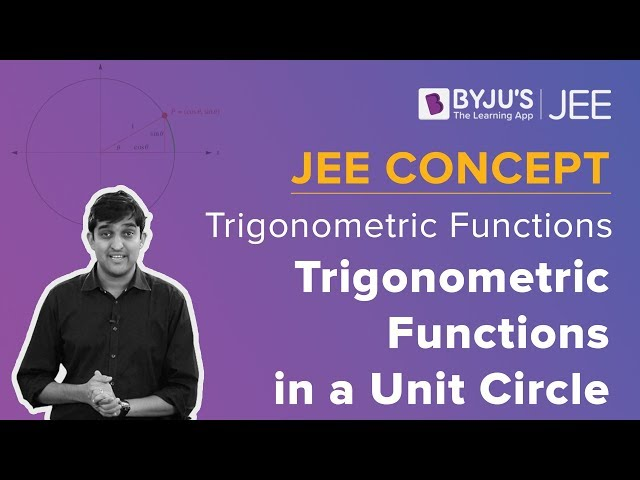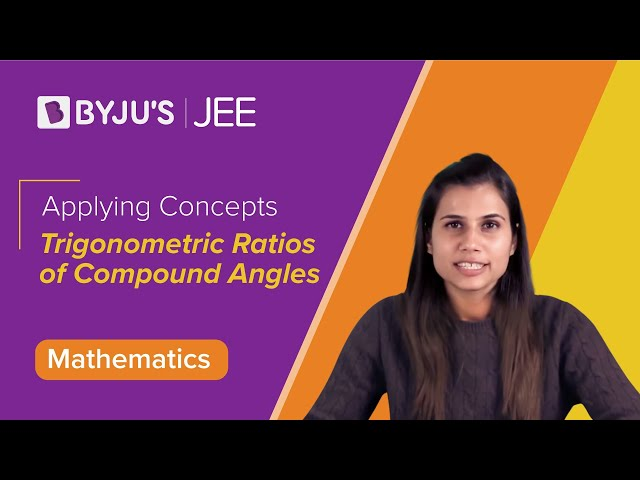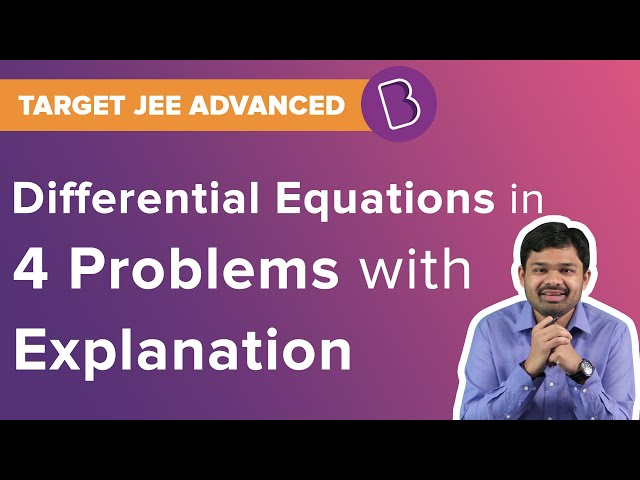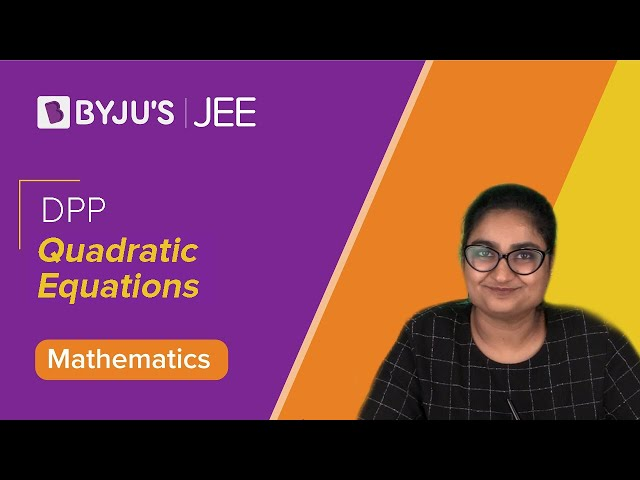Win up to 100% scholarship on Aakash BYJU'S JEE/NEET courses with ABNAT Win up to 100% scholarship on Aakash BYJU'S JEE/NEET courses with ABNAT

# JEE Main 2020 Maths Paper With Solutions Shift 2 Sept 5

JEE Main 2020 Maths paper September 5 Shift 2 solutions are available on this page. These are prepared by specialized experts at BYJU’S. Practising previous question papers is one of the best methods for JEE preparation. Students are recommended to revise and learn these solutions so that they can easily crack the JEE Main exam. Students can download these solutions in PDF format for free.

## JEE Main 2020 Maths Paper With Solutions Shift 2 September 5

September 5 Shift 2 – Maths

1. If x = 1 is a critical point of the function f(x) = (3x2+ax-2-a)ex, then:

1) x = 1 is a local minima and x = -2/3 is a local maxima of f.
2) x = 1 is a local maxima and x = -2/3 is a local minima of f.
3) x = 1 and x = -2/3 are local minima of f.
4) x = 1 and x = -2/3 are local maxima of f.

f(x) = (3x2+ax-2-a)ex

f’(x) = (3x2+ax-2-a)ex +(6x+a)ex = 0

ex [3x2+(a+6) x-2] =0

at x = 1, 3+a+6-2 = 0

a = -7

f(x) = (3x2-7x+5)ex

f’(x) = (6x-7)ex +(3x2-7x+5)ex

= ex(3x2-x-2) = 0

= 3x2-3x+2x-2 = 0

= (3x+2)(x-1) = 0

x = 1, -2/3

x = 1 is point of local minima.

x = –2/3 is point of local maxima.

2.

$$\begin{array}{l}\lim _{x \rightarrow 0} \frac{x\left(e^{\left(\sqrt{1+x^{2}+x^{4}}-1\right) / x}-1\right)}{\sqrt{1+x^{2}+x^{4}}-1}\end{array}$$

1) is equal to √e
2) is equal to 1
3) is equal to 0
4) does not exist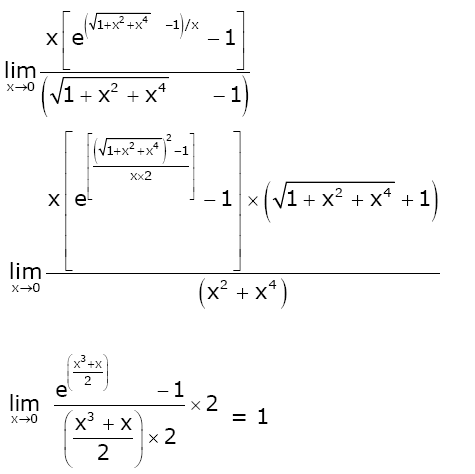3. The statement (p → (q → p)) → (p → (p ˅ q)) is:

1) equivalent to (p˅q)˄ (~ p)
2) equivalent to (p˄q)˅(~ p)
4) a tautology

 p q q→ p p→( q→ p) p˅q p → (p˅q) (p→( q→ p)) → (p → (p ˅ q)) T T T T T T T T F T T T T T F T F T T T T F F T T F T T

4. If L = sin2(π/16) – sin2(π/8) and M = cos2(π/16) – sin2(π/8), then

1) M = (1/2√2)+(1/2)cos (π/8)
2) M = (1/4√2)+(1/4)cos (π/8)
3) L = -(1/2√2)+(1/2)cos (π/8)
4) L = (1/4√2)-(1/4)cos (π/8)

L = sin(3π/16) sin(-π/16)

L = (-1/2)[cos(π/8)-cos(π/4)]

L = (1/2√2)-(1/2)cos (π/8)

M = cos(3π/16)cos(π/16)

M = (1/2)[cos(π/4)+cos(π/8)]

M = (1/2√2)+(1/2)cos(π/8)

5. If the sum of the first 20 terms of the series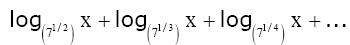is 460, then x is equal to:

1) 71/2
2) 72
3) e2
4) 746/21

(2+3+4+…+21)log7x = 460

⇒ (20×(21+2)/2)log7 x = 460

230 log7x = 460

log7x = 2

x = 72

6. There are 3 sections in a question paper and each section contains 5 questions. A candidate has to answer a total of 5 questions, choosing at least one question from each section. Then the number of ways, in which the candidate can choose the questions, is:

1) 2250
2) 2255
3) 1500
4) 3000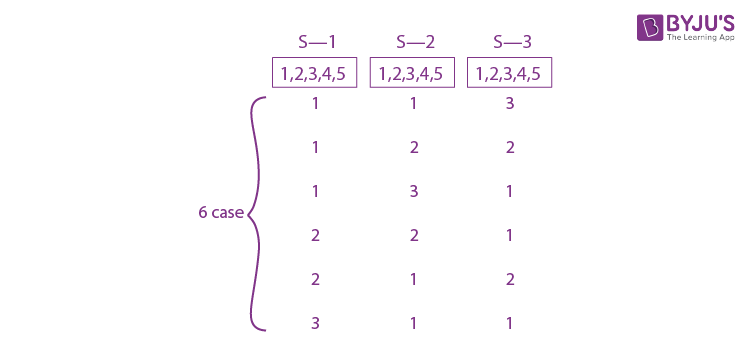3(5C1×5C2×5C2)+3(5C1 ×5C1×5C3)

= 3(5×5×2×5×2)+3(5×5×10)

= 750 + 1500

= 2250

7. If the mean and the standard deviation of the data 3,5,7,a,b are 5 and 2 respectively, then a and b are the roots of the equation:

1) x2-20x+18 = 0
2) x2-10x+19 = 0
3) 2x2-20x+19 = 0
4) x2-10x+18 = 0

S.D =

$$\begin{array}{l}\sqrt{\frac{\sum x_{i}^{2}}{n}-(\bar{x})^{2}}\end{array}$$

22 = (83+a2+b2)/5 -(5)2

4 = (83+a2+b2)/5 -(25)

29×5-83 = a2+b2

⇒ a2+b2 = 62

(a+b+15)/5 = 5

⇒ a+b = 10 ..(i)

2ab = 100-62 = 38

ab = 19 ..(ii)

from (i) and (ii)

x2-10x+19 = 0

8. The derivative of tan-1(√(1+x2)-1)/x with respect to tan-1(2x√(1-x2))/(1-2x2)) at x = 1/2 is:

a) 2√3/3
b) 2√3/5
c) √3/12
d) √3/10

x = tan θ

u = tan-1(sec θ-1)/tan θ

= tan-1 (tan θ/2)

= θ/2

= (tan-1 x)/2

x = sin θ

v = tan-1(2 sinθ cosθ/cos 2θ) = 2θ

= 2 sin-1x

du/dv = ½(1+x2)×√(1-x2)/2

= (√3/2×2)×(4/5×2)

= √3/10

9. If ∫ cos θ/(5+7sinθ-2+2sin2θ)dθ = A loge |B(θ)| + C where C is a constant of integration, then B(θ)/A can be:

1) 5(2sinθ+1)/(sinθ+3)
2) 5(sinθ+3)/(2sinθ+1)
3) (2sinθ+1)/(sinθ+3)
4) (2sinθ+1)/5(sinθ+3)

∫ cos θ/(5+7sinθ-2+2sin2θ)dθ

Put sinθ = t, cosθ dθ = dt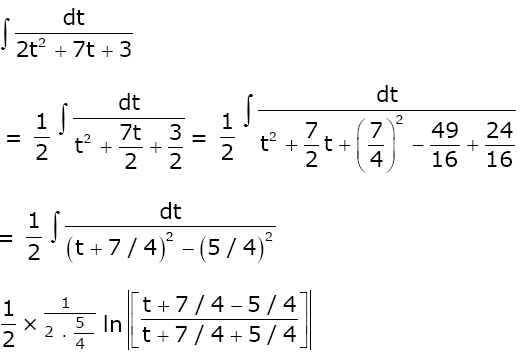= (1/5)ln |(sinθ+1/2)/(sinθ+3)|+C

B(θ)/A = 5(2sinθ+1)/(sinθ+3)

10. If the length of the chord of the circle, x2+y2 = r2(r>0) along the line, y-2x = 3 is r, then r2 is equal to:

1) 12
2) 24/5
3) 9/5
4) 12/5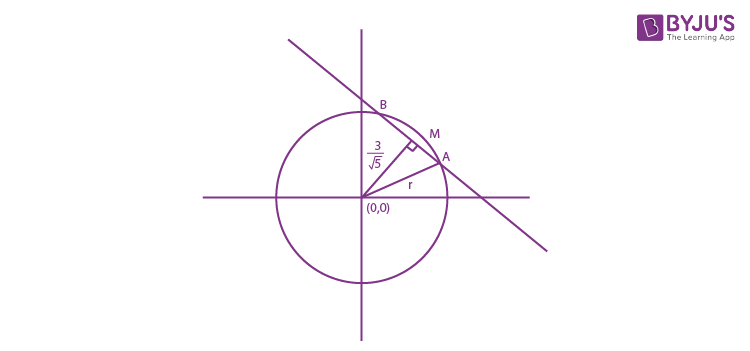AB = 2√(r2-9/5) = r

r2-9/5 = r2/4

3r2/4 = 9/5

r2 = 12/5

11. If α and β are the roots of the equation, 7x2-3x-2 = 0, then the value of α/1-α2 and β/1-β2 is equal to:

1) 27/32
2) 1/24
3) 27/16
4) 3/8

α+β = 3/7

αβ = -2/7

[(α+β)-αβ(α+β)]/[1-(α22)+(αβ)2]

= [(3/7)+(2/7)×(3/7)](1-{(9/49)+(4/7)}+(4/49))

= (21+6)/49(16/49)

= 27/16

12. If the sum of the second, third and fourth terms of a positive term G.P. is 3 and the sum of its sixth, seventh and eighth terms is 243, then the sum of the first 50 terms of this G.P. is:

1) (2/13)(350-1)
2) (1/26)(349-1)
3) (1/13)(350-1)
4) (1/26)(350-1)

(ar+ar2+ar3)/(ar5+ar6+ar7) = 3/243

(1+r+r2)/r4(1+r+r2) = 1/81

r = 3

a(3+9+27) = 3

a = 3/39 = 1/13

S50 = a(r50-1)/(r-1)

= 1/13(350-1)/2

= (1/26)(350-1)

13. If the line y = mx+c is a common tangent to the hyperbola (x2/100)-(y2/64) = 1 and the circle x2+y2 = 36, then which one of the following is true?

1) 4c2 = 369
2) c2 = 369
3) 8m+5 = 0
4) 5m = 4

c = √(a2m2-b2)

c = √(100m2-64)

y = mx√(100m2-64)

d(0,0) = 6

|√(100m2-64)/√(m2+1)| = 6

100m2 -64 = 36m2+36

64m2 = 100

m = 10/8

c2 = 100×(100/64)-64

⇒ 164×36/64

4c2 = 369

14. The area (in sq. units) of the region A = {(x,y): (x-1)[x] ≤y ≤2√x, 0 ≤x ≤2} where [t] denotes the greatest integer function, is:

1) (4/3)√2-(1/2)
2) (8/3)√2-(1/2)
3) (8/3)√2-1
4) (4/3)√2+1

y = f(x) = (x-1) [x]

=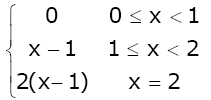y2 ≤ 4x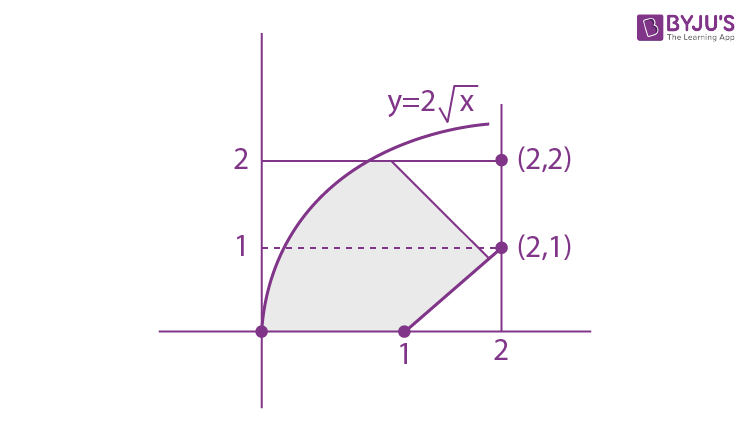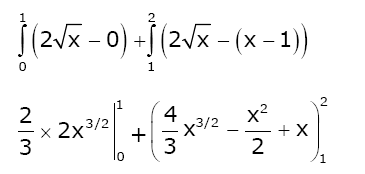= (4/3)+{(4/3)×2√2-2+2)-((4/3)+(1/2))}

= (4/3)+(8√2/3)-(4/3)-(1/2)

= (8√2/3)-(1/2)

Solution: (2)

15. If a+x = b+y = c+z+1, where a,b,c,x,y,z are non-zero distinct real numbers, then

$$\begin{array}{l}\begin{vmatrix} x &a+y & x+a\\ y & b+y & y+b\\ z & c+y & z+c \end{vmatrix}\end{array}$$
is equal to:

1) y(a-b)
2) 0
3) y(b-a)
4) y(a-c)

Given a+x = b+y = c+z+1

Now,

$$\begin{array}{l}\begin{vmatrix} x &a+y & x+a\\ y & b+y & y+b\\ z & c+y & z+c \end{vmatrix}\end{array}$$

=

$$\begin{array}{l}\begin{vmatrix} x &a+y & a\\ y & b+y & b\\ z & c+y & c \end{vmatrix}\end{array}$$
(C3 → C3-C1)

=

$$\begin{array}{l}\begin{vmatrix} x &y & a\\ y & y & b\\ z & y & c \end{vmatrix}\end{array}$$
(C2 → C2-C3) =
$$\begin{array}{l}y\begin{vmatrix} x &1 & a\\ y & 1 & b\\ z & 1 & c \end{vmatrix}\end{array}$$

R2 → R2 – R1 and R3 → R3 – R1

=

$$\begin{array}{l}y\begin{vmatrix} x &1 & a\\ y-x & 0 & b-a\\ z-x & 0 & c-a \end{vmatrix}\end{array}$$

= y[x×0-1{(y-x)(c-a)-(b-a)(z-x)}+a×0]

= y[bz-bx-az+ax-(cy-ay-cx+ax)]

= y[bz-bx-az-cy+ay+cx]

= y[b(z-x)+a(y-z)+c(x-y)]

= y[b{a-c-1}+a(c-b+1)+c(b-a)]

= y[ab-bc-b+ac-ab+a+bc-ac]

= y(a-b)

16. If for some α∈ R , the lines L1 : (x+1)/2 = (y-2)/-1 = (z-1)/1 and L2 : (x+2)/α = (y+1)/(5-α) = (z+1)/1 are coplanar, then the line L2 passes through the point:

1) (2, -10, -2)
2) (10, -2, -2)
3) (10, 2, 2)
4) (-2, 10, 2)

A(-1,2,1), B(-2,-1,-1)

$$\begin{array}{l}\left [ \overrightarrow{AB} \; \overrightarrow{b_{1}} \; \overrightarrow{b_{2}} \right ]=0\end{array}$$
$$\begin{array}{l}\begin{vmatrix} -1 &-3 &-2 \\ 2& -1 & 1\\ \alpha & 5-\alpha & 1 \end{vmatrix}=0\end{array}$$

-1(-1+α-5)+3(2-α)-2(10-2α+α) = 0

6-α+6-3α+2α-20 = 0

-8-2α = 0

α = -4

L2: (x+2)/-4 = (y+1)/9 = (z+1)/1

Check options. (2, -10, -2) satisfies above equation.

17. The value of [((-1+i√3)/(1-i))]30 is:

1) 215i
2) -215
3) -215i
4) 65

[((-1+i√3)/(1-i))]30 ⇒ [((-1+i√3)/2)(1+i)]30

ω30(1+i)30 = 215(-i)

18. Let y = y(x) be the solution of the differential equation cos x (dy/dx)+2y sinx = sin2x, x∈(0, π/2). If y(π/3) = 0, then y(π/4) is equal to:

1) 2+√2
2) √2-2
3) (1/√2)-1
4) 2-√2

(dy/dx) +(2tanx) y = 2sinx

I.F = e2ln(sec x) = sec2x

y(sec2x) = 2∫sinx/cos2x dx

= 2∫secx tan x dx

= 2 secx+C

y(π/3) = 0

0 = 2×2+c

⇒ c = -4

y(sec2x) = 2 secx-4

x = π/4

2y = 2√2-4

y = √2-2

19. If the system of linear equations

x+y+3z = 0

x+3y+k2z = 0

3x+y+3z = 0

has a non-zero solution (x,y,z) for some k∈R, then x+(y/z) is equal to:

1) -9
2) 9
3) -3
4) 3

$$\begin{array}{l}\begin{vmatrix} 1 & 1 & 3\\ 1& 3& k^{2}\\ 3& 1 & 3 \end{vmatrix}=0\end{array}$$

(9-k2)-(3-3k2) + 3(-8)=0

9-k2-3+3k2-24 = 0

2k2-18 = 0

k2 = 9

k = 3, -3

x+y+3z = 0 ..(i)

x+3y+9z = 0 ..(ii)

Equation (ii)-(i)

2y +6z= 0

y = -3z

y/z = -3

2x = 0

x = 0

x+(y/z) = -3

20. Which of the following points lies on the tangent to the curve 4x3ey+x4ey+2√(y+1) = 3 at the point (1,0)?

1) (2,6)
2) (2,2)
3) (-2,6)
4) (-2,4)

4x3ey+x4eyy’ +2y’/2√(y+1) = 0

At (1,0)

4+y’+(2y’/2) = 0

2y’ = -4

⇒ y’ = -2

E.O.T.:

y = -2(x-1)

2x+y = 2

Check options given.

(-2,6) satisfies the equation 2x+y = 2.

21. Let A = {a,b,c} and B = {1,2,3,4}. Then the number of elements in the set C = {f : A → B 2∈ f(A)and f is not one-one} is:

C = {f : A → B 2 ∈ f(A) and f is not one-one}

Case-I : If f(x) = 2 ∀ x∈ A, then number of function = 1

Case-II : If f(x) = 2 for exactly two elements then total number of many-one function

= 3C2 3C1 = 9

Case -III : If f(x) = 2 for exactly one element then total number of many-one function

= 3C1 3C1 = 9

Total = 19

22. The coefficient of x4 in the expansion of (1+x+x2+x3)6 in powers of x, is:

(1+x+x2+x3)n = (1+x)n(1+x2)n

(1+x+x2+x3)6= (1+x)6(1+x2)6

Coefficient of x4 nC0 nC2+ nC2. nC1+ nC4. nC6

= 6C0 6C2+ 6C2. 6C1+ 6C4. 6C6

= 15+(15×6)+15

= 120

23. Let the vectors

$$\begin{array}{l}\vec{a}\end{array}$$
,
$$\begin{array}{l}\vec{b}\end{array}$$
,
$$\begin{array}{l}\vec{c}\end{array}$$
such that
$$\begin{array}{l}\left |\vec{a} \right | = 2\end{array}$$
,
$$\begin{array}{l}\left |\vec{b} \right | = 4\end{array}$$
, and
$$\begin{array}{l}\left |\vec{c} \right | = 4\end{array}$$
. If the projection of vector b on vector a is equal to the projection of vector c on vector a and b is perpendicular to vector c, then the value of
$$\begin{array}{l}\left |\vec{a} + \vec{b}-\vec{c}\right |\end{array}$$
is :

$$\begin{array}{l}\frac{\vec{b}.\vec{a}}{2}= \frac{\vec{c}.\vec{a}}{2}\end{array}$$

$$\begin{array}{l}\vec{b}.\vec{a}= \vec{c}.\vec{a}\end{array}$$

$$\begin{array}{l}\vec{b}.\vec{c}= 0\end{array}$$

$$\begin{array}{l}\left |\vec{a} + \vec{b}-\vec{c}\right |\end{array}$$
= √(a2+b2+c2 +2a.b-2b.c-2a.c)

= √(4+16+16)

= 6

24. If the lines x+y = a and x-y = b touch the curve y = x2-3x+2 at the points where the curve intersects the x-axis, then a/b is equal to: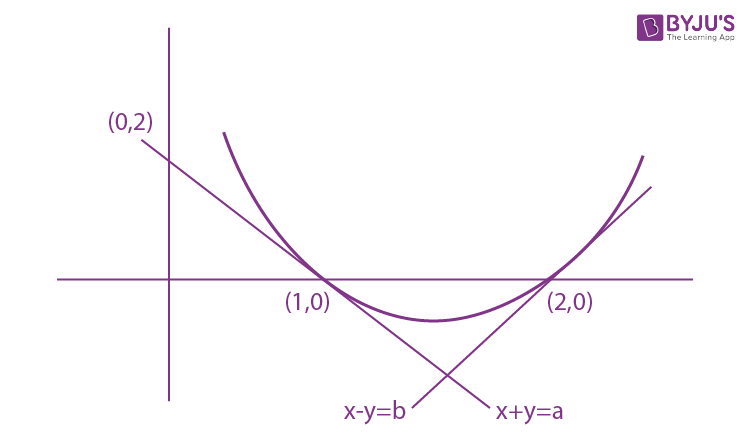y -0 = -1(x-1)

x+y = 1

⇒ a = 1

y-0 = x-2

x-y = 2 = b = 2

a/b = 1/2

25. In a bombing attack, there is 50% chance that a bomb will hit the target. At least two independent hits are required to destroy the target completely. Then the minimum number of bombs, that must be dropped to ensure that there is at least 99% chance of completely destroying the target, is

Let n is total no. of bombs being dropped

at least 2 bombs should hit

⇒ prob ≥ 0.99

p(x≥2) ≥0.99

1-p(x<2) ≥ 0.99

1-p(x=0)+p(x=1) ≥ 0.99

1-[nC0(p)0qn+nC1(p)1(q)n-1] ≥ 0.99

1-[qn+pnqn-1] ≥0.99

1-[(1/2n)+(n/2)×(1/2n-1)] ≥0.99

1-(1/2n)(n+1)≥ 0.99

0.01 ≥ (1/2n)(n+1)

2n ≥100+100n

n ≥ 11